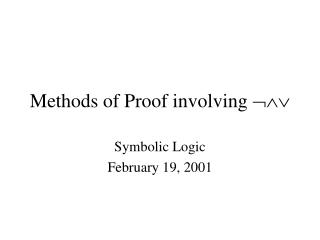Download PresentationMethods of Proof involving Methods of Proof involving  - PowerPoint PPT Presentation

Download PresentationMethods of Proof involving 
An Image/Link below is provided (as is) to download presentation

Download Policy: Content on the Website is provided to you AS IS for your information and personal use and may not be sold / licensed / shared on other websites without getting consent from its author. While downloading, if for some reason you are not able to download a presentation, the publisher may have deleted the file from their server.

- - - - - - - - - - - - - - - - - - - - - - - - - - - E N D - - - - - - - - - - - - - - - - - - - - - - - - - - -
Presentation Transcript

1. Methods of Proof involving  Symbolic Logic February 19, 2001

2. Outline • Valid Steps • Logical Truth • Conjunction Elimination • Conjunction Introduction • Disjunction Introduction • Proof by Cases • Negation Introduction

3. Logical Truth • If a sentence is a logical truth, it is always true, no matter what. • Since it is always truth, you are allowed to assert it without any other justification. • We have seen this already with Reflexivity of Identity. • Because a = a is a logical truth, you can introduce it at any time.

4. Examples • a = a (because an object is always identical with itself (def. of identity)) • P  P (because of the truth table for ) • (P  P) (because of the truth table for )

5. Conjunction Elimination/Simplification • If we know P  Q, we also know P (as well as Q). • Thus, 1. P  Q 2. P 1, Conjunction Elimination and, 1. P  Q 2. Q 1, Conjunction Elimination

6. Conjunction Introduction • The converse is true as well. • If we know P and we know Q, we know P  Q. So, 1. P 2. Q 3. P  Q 1, 2 Conjunction Intro.

7. Disjunction Introduction/Addition • Because of the truth table for , we know that a disjunction is true if one of its disjuncts is true. • This allows us to introduce anything we want using a disjunction. So, given P, we can deduce P  Q P  (Q  R) P  ((M  S)  (T  (Q  R)))

8. Disjunction Introduction/Addition • In English, we know that if “It is President’s Day” is true, “Either it is President’s Day or frogs will fly out of my butt” is true as well. • So, 1. P 2. P  Q 1 Disjunction Intro.

9. Proof by Cases/ Disjunction Elimination • Suppose we know that (P  Q)  (P  R) • In order for this disjunction to be true, one or the other sides must be true. • Since P is true in both cases (by conjunction elimination), P must be true. So, since • P  Q implies P and, • P  R also implies P, • P must be true no matter what.

10. Proof by Contradiction/Reductio ad absurdum or Negation Intro. • Writers and speakers who want to disprove a claim often assume the claim and then show how it leads to a contradiction. • If the claim or set of claims leads to a contradiction, it cannot possibly be true. • We can use this technique in more formal situations as well.

11. Negation Introduction • Consider, 1. Either Max is a Cat or Max is an Owl 2. Sofie is an Aardvark 3. Assume Max = Sofie If Max = Sofie, then Either Sofie is a Cat or Sofie is an Owl (1, 3 Ind. Id.) Sofie can’t be either a Cat or an Owl, since Sofie is an Aardvark (2) Therefore Max  Sofie

12. Contradictory Premises • Note that 1. P  P 2. R • Since P  P cannot possibly be true, if we assert that it is true, it implies every possible state of affairs.

13. Homework Note • You don’t have to do problems 28, 37, or 38 for the next homework set (or ever). • Because this isn’t a math class, we will not have problems like this on the test.